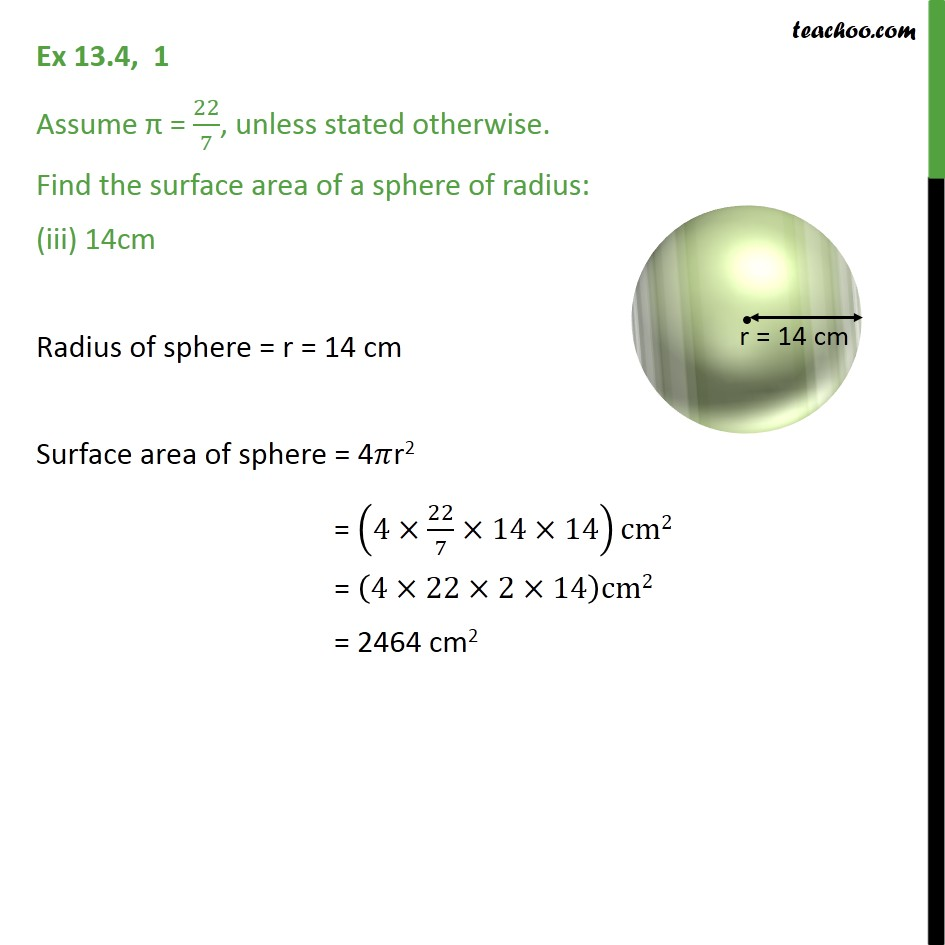Ex 11.2

Chapter 11 Class 9 Surface Areas and Volumes
Serial order wiseLearn in your speed, with individual attention - Teachoo Maths 1-on-1 Class

### Transcript

Ex 11.2, 1 Assume = 22/7, unless stated otherwise. Find the surface area of a sphere of radius: (iii) 14cm Radius of sphere = r = 14 cm Surface area of sphere = 4 r2 = (4 22/7 14 14)cm2 = (4 22 2 14)cm2 = 2464 cm2